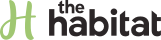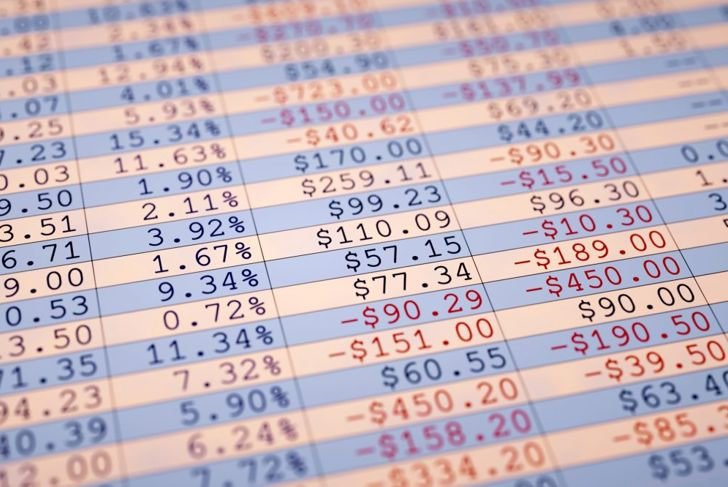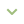Home# How to Find the Mean

Sara AndersonThe arithmetic mean, also known by many as the average, is a number often used in normal day-to-day life. Teachers use it to calculate grades, workers employ it to determine the average amount that they bring home each month, and meteorologists can utilize it to measure the average daily temperature for a month. In simple terms, the mean is simply the total amount of a set of numbers divided by the number of values used. Calculating the mean is not difficult, even when dealing with a large number of values.

01

## Basic Mean CalculationThe basic calculation of the mean involves collecting a series of numbers, whether it is a collection of test scores or the time it takes to travel from one side of town to the other on different days of the week. Once the numbers are added together, they are multiplied by the number of values provided. For travel times during the work week, people should find five separate values, meaning that five becomes the number that the total is divided by. In mathematical terms, the mean = (a+b+c...)/(total number of entries).

PeopleImages / Getty Images

02

## Basic Mean Example ProblemFotografiaBasica / Getty Images

Finding the average or mean of a group of numbers is straightforward. Here is a group of numbers: 15, 31, 39, 50, 32, 42. What is the average of this collection of numbers? The first step is to count the number of values. There are six numbers, so six will be the number on the bottom of a division problem. Now, to add the values together.

15+31+39+50+33+42 = 210

So, following the division formula, students should realize that 210 represents the total of all the numbers added (the top value of the formula). Inserting the numbers:

210/6 = 35

The average value of these six numbers is 35.

03

## Manipulating Numbers to Solve the Meanleventince / Getty Images

In some cases, it is possible to simplify the computation. Take the following numbers as an example: 20, 40, 30, 50. It is possible to add all the numbers together and divide by 4 (the number of values present). However, it is also possible to use common sense to lower the amount of computation needed. It is easy to figure out the average of 20 and 40 (A):

A. (20 +40) / 2 = 60/2 = 30

And then figure out the average between 30 and 50 (B):

B. (30 +50) / 2 = 80/2 = 40

Then we figure out the average between these two answers, A and B:

(30 + 40) / 2 = 70/2 = 35.

Therefore, the average of the four numbers provided is 35. Following the formula will garner the same answer, but students may need a calculator:

(20+30+40+50) /4 = 140/4 = 35

04

## Rounding and Being Exactmarekuliasz / Getty Images

It is uncommon in most cases for an average or mean number to be a whole number. Often, the average has a remainder or decimal component. For example, consider the following set of numbers: 40, 35, 28, 24. Computing the mean involves adding the numbers of the set together (40+35+28) and dividing by 3 (the total numbers in the set).

(42+36+28) / 3 = 103/ 3 = 34.33333333333

In this case, students should round the number as requested by the instructors. Often, they will be asked to round to the nearest whole number. In this case, the average/mean of this set of numbers is 34.

05

## Using a Frequency ChartMicrovOne / Getty Images

Frequency tables are used when calculating the mean of a large number of values. This is particularly useful when numbers repeat. Imagine that a class has ten students who take the same exam. The only possible scores are 10, 20, and 30. It would be difficult to add ten separate scores to find the average. Instead, students can use the repeating values to simplify the problem. For example, 3 students receive a score of 10, 6 students receive a score of 20 and 1 receives a 30. Here is what the problem would look like:

(10 x 3 ) + (20 x 6) + (30 x 1 ) = 30 + 120 + 30 = 180

Using this number as the total, it can be divided by 10 (the total number of students taking the test):

180/10 = 18

Therefore, the mean score of all students is 18.

06

## Connection Between Mean, Median, and ModeMiddle school students often work with mean, median and mode at the same time. This is because the three concepts intertwine, and in fact are all considered "averages." They all deal with groups of numbers. The mean is the average of all the numbers added together; the median is the middle value of the numbers (when written from lowest to highest it is the "middle" number. The mode is the number value that occurs most frequently. Some sets don't have a mode.

lolostock / Getty Images

07

## The Mean of Negative Numbersmatejmo / Getty Images

Figuring out the mean of a set of numbers can be complicated if any of the numbers are negative. The steps to solving for the mean, however, is the same as if all the numbers were positive. Students should add the numbers together and divide by the total number of values. For example, the following set of five numbers includes both negative and positive values:

(-4, 2, 6, -1, 7)

Adding these numbers together results in the following:

-4 + 2 + 6 + -1 + 7 = 10

Now, divide this result by the number of values in the set (5):

10 / 5 = 2

The mean of this set of numbers is 2.

08

## Financial Uses for the MeanMinerva Studio / Getty Images

Those working on a budget will appreciate knowing how to find the mean, as it can help to determine how much money their phone bill averages per month or what they usually spend on groceries. Say a family of four wants to go to Myrtle Beach and plans to save up money using "eating out" funds. A hotel for three nights will cost \$500. Using the last three months' receipts, they realize that they have spent \$137, \$95, and \$267 on dining. How many months will it take for them to save up the money for the hotel?

To solve, take the three values and add together:

137 + 95 + 267 = 499

Divide by the number of values (3) = 499 / 3 = 166.33333 or \$166. With this average, it will take the family \$500/\$166 = 3, or 3 months to save up money for a vacation.

09

## Solving a Murder Using the MeanIt might be unusual to think of finding a mean to solve a murder, but it has been done in real crime cases. It is particularly useful to test a suspect's alibi. Imagine that a suspect claims to have been running errands across town during the time that police believe a person was killed. They can prove their timeline most of the day but have a 45-minute window where they cannot prove where they were. Detectives can drive the route from where they claim to have been to the crime scene. To be scientifically accurate they drive different routes at different times and then take the average of the different travel times. If the average of the times taken is less than 45 minutes, the suspect could have committed the murder.

Prathaan / Getty Images

10

## Other Types of MeansWhile the arithmetic mean is the most commonly referred to, other means can be used by people to determine statistical averages. A geometric mean is often used by investors to calculate their return on investments. It is calculated using logarithms and gives a more accurate result than the traditional average. The harmonic mean can be used to compare speeds or other measures that include a unit measurement and allow users to discount anomalies in the measurements, such as one low score in a group of test-takers. Again, it is used to increase the accuracy of the measurement. Students use reciprocals to compute the harmonic mean.

gopixa / Getty Images